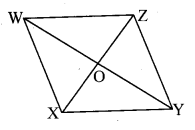PRINTABLE FOR KIDS

XII (12) HSC

XI (11) FYJC
X (10) SSC

### Balbharati solutions for Mathematics 8th Standard Maharashtra State Board chapter 8 - Quadrilateral : Constructions and Types [Latest edition]

PRACTICE SET 8.1 [PAGE 43]

### Balbharati solutions for Mathematics 8th Standard Maharashtra State Board Chapter 8 Quadrilateral : Constructions and Types Practice Set 8.1 [Page 43]

Practice Set 8.1 | Q 1 | Page 43

Construct the following quadrilateral of given measures.

In ☐ MORE, l(MO) = 5.8 cm, l(OR) = 4.4 cm, m∠M = 58°, m∠O = 105°, m∠R = 90° .

Practice Set 8.1 | Q 2 | Page 43

Construct the following quadrilateral of given measures.

Construct ☐ DEFG such that l(DE) = 4.5 cm, l(EF) = 6.5 cm, l(DG) = 5.5 cm, l(DF) = 7.2 cm, l(EG) = 7.8 cm.

Practice Set 8.1 | Q 3 | Page 43

Construct the following quadrilateral of given measures.

In ☐ ABCD l(AB) = 6.4 cm, l(BC) = 4.8 cm, m∠A = 70° , m∠B = 50° , m∠C = 140°.

Practice Set 8.1 | Q 4 | Page 43

Construct the following quadrilateral of given measures.

Construct ☐ LMNO such that l(LM) = l(LO) = 6 cm, l(ON) = l(NM) = 4.5 cm, l(OM) = 7.5 cm.

PRACTICE SET 8.2 [PAGES 46 - 47]

### Balbharati solutions for Mathematics 8th Standard Maharashtra State Board Chapter 8 Quadrilateral : Constructions and Types Practice Set 8.2 [Pages 46 - 47]

Practice Set 8.2 | Q 1 | Page 46

Draw a rectangle ABCD such that l(AB) = 6.0 cm and l (BC) = 4.5 cm.

Practice Set 8.2 | Q 2 | Page 46

Draw a square WXYZ with side 5.2 cm.

Practice Set 8.2 | Q 3 | Page 46

Draw a rhombus KLMN such that its side is 4 cm and m∠K = 75° .

Practice Set 8.2 | Q 4 | Page 46

If diagonal of a rectangle is 26 cm and one side is 24 cm, find the other side.

Practice Set 8.2 | Q 5 | Page 47

Lengths of diagonals of a rhombus ABCD are 16 cm and 12 cm. Find the side and perimeter of the rhombus.

Practice Set 8.2 | Q 6 | Page 47

Find the length of diagonal of a square with side 8 cm .

Practice Set 8.2 | Q 7 | Page 47

Measure of one angle of a rhombus is 50° .find the measures of remaining three angles.

PRACTICE SET 8.3 [PAGES 49 - 50]

### Balbharati solutions for Mathematics 8th Standard Maharashtra State Board Chapter 8 Quadrilateral : Constructions and Types Practice Set 8.3 [Pages 49 - 50]

Practice Set 8.3 | Q 1 | Page 49

Measures of opposite angles of a parallelogram are (3x − 2)° and (50 − x)° . Find the measure of its each angle.

Practice Set 8.3 | Q 2 | Page 50

Referring the adjacent figure of a parallelogram, write the answer of questions given below.(1) If l(WZ) = 4.5 cm then l(XY) = ?
(2) If l(YZ) = 8.2 cm then l(XW) = ?
(3) If l(OX) = 2.5 cm then l(OZ) = ?
(4) If l(WO) = 3.3 cm then l(WY) = ?
(5) If m∠WZY = 120° then m∠WXY = ? and m∠XWZ = ?

Practice Set 8.3 | Q 3 | Page 50

Construct a parallelogram ABCD such that l(BC) = 7 cm, m∠ABC = 40° , l(AB) = 3 cm.

Practice Set 8.3 | Q 4 | Page 50

Ratio of consecutive angles of a quadrilateral is 1:2:3:4. Find the measure of its each angle. Write, with reason, what type of a quadrilateral it is.

Practice Set 8.3 | Q 5 | Page 50

Construct ☐ BARC such that l(BA) = l(BC) = 4.2 cm, l(AC) = 6.0 cm, l(AR) = l(CR) = 5.6 cm

Practice Set 8.3 | Q 6 | Page 50

Construct ☐ PQRS, such that l(PQ) = 3.5 cm, l(QR) = 5.6 cm, l(RS) = 3.5 cm, m∠Q = 110° , m∠R = 70° . If it is given that ☐ PQRS is a parallelogram, which of the given information is unnecessary?

## PDF FILE TO YOUR EMAIL IMMEDIATELY PURCHASE NOTES & PAPER SOLUTION. @ Rs. 50/- each (GST extra)

SUBJECTS

HINDI ENTIRE PAPER SOLUTION

MARATHI PAPER SOLUTION
SSC MATHS I PAPER SOLUTION
SSC MATHS II PAPER SOLUTION
SSC SCIENCE I PAPER SOLUTION
SSC SCIENCE II PAPER SOLUTION
SSC ENGLISH PAPER SOLUTION
SSC & HSC ENGLISH WRITING SKILL
HSC ACCOUNTS NOTES
HSC OCM NOTES
HSC ECONOMICS NOTES
HSC SECRETARIAL PRACTICE NOTES

2019 Board Paper Solution

HSC ENGLISH SET A 2019 21st February, 2019

HSC ENGLISH SET B 2019 21st February, 2019

HSC ENGLISH SET C 2019 21st February, 2019

HSC ENGLISH SET D 2019 21st February, 2019

SECRETARIAL PRACTICE (S.P) 2019 25th February, 2019

HSC XII PHYSICS 2019 25th February, 2019

CHEMISTRY XII HSC SOLUTION 27th, February, 2019

OCM PAPER SOLUTION 2019 27th, February, 2019

HSC MATHS PAPER SOLUTION COMMERCE, 2nd March, 2019

HSC MATHS PAPER SOLUTION SCIENCE 2nd, March, 2019

SSC ENGLISH STD 10 5TH MARCH, 2019.

HSC XII ACCOUNTS 2019 6th March, 2019

HSC XII BIOLOGY 2019 6TH March, 2019

HSC XII ECONOMICS 9Th March 2019

SSC Maths I March 2019 Solution 10th Standard11th, March, 2019

SSC MATHS II MARCH 2019 SOLUTION 10TH STD.13th March, 2019

SSC SCIENCE I MARCH 2019 SOLUTION 10TH STD. 15th March, 2019.

SSC SCIENCE II MARCH 2019 SOLUTION 10TH STD. 18th March, 2019.

SSC SOCIAL SCIENCE I MARCH 2019 SOLUTION20th March, 2019

SSC SOCIAL SCIENCE II MARCH 2019 SOLUTION, 22nd March, 2019

XII CBSE - BOARD - MARCH - 2019 ENGLISH - QP + SOLUTIONS, 2nd March, 2019

HSC Maharashtra Board Papers 2020

(Std 12th English Medium)

HSC ECONOMICS MARCH 2020

HSC OCM MARCH 2020

HSC ACCOUNTS MARCH 2020

HSC S.P. MARCH 2020

HSC ENGLISH MARCH 2020

HSC HINDI MARCH 2020

HSC MARATHI MARCH 2020

HSC MATHS MARCH 2020

SSC Maharashtra Board Papers 2020

(Std 10th English Medium)

English MARCH 2020

HindI MARCH 2020

Hindi (Composite) MARCH 2020

Marathi MARCH 2020

Mathematics (Paper 1) MARCH 2020

Mathematics (Paper 2) MARCH 2020

Sanskrit MARCH 2020

Important-formula

THANKS